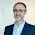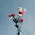## Friday, 8 July 2016

### Time Averages of NetCDF files from ECMWF in ArcGIS with R-Bridge

With this post I would like to talk again about R-Bridge, which allows a direct communication between ArcGIS and R.
In the previous post, I presented a very simple application of R-Bridge where I built a toolbox to perform k-means clustering on point shapefiles. The purpose of that post was to explain the basics of the technology, but its scientific scope was limited. However, I would like to try step by step to translate more of my R scripts in ArcGIS, so that more people can use them even if they are not experts in R.
In this post I will start presenting a toolbox to handle NetCDF files downloaded from the European Centre for Medium-Range Weather Forecasts (ECMWF).

ECMWF
The European Centre for Medium-Range Weather Forecasts provides free access to numerous weather data through their website. You can go directly to this page to take a look at the data available: http://www.ecmwf.int/en/research/climate-reanalysis/browse-reanalysis-datasets
The data are freely accessible and downloadable (for research purposes!!) but you need to register to the website to be able to do so.

My Research
For a research I'm doing right now I downloaded the ERA Interim dataset from 2010 to 2015 from this access page: http://apps.ecmwf.int/datasets/data/interim-full-daily/levtype=sfc/

The data are provided in large NetCDF files, which include all the weather variables I selected and for the entire time frame. In R, NetCDF files can be easily imported, using the packages `raster` and `ncdf4`, as a raster brick. This will have a X and Y dimensions, plus a time dimension for each of the variables I decided to download. Since I wanted 5 years and the ERA dataset include four datasets per day, I had quite a lot of data.
I decided that for the research I was planning to do I did not need each and every one of these rasters, but rather some time averages, for example the monthly averages for each variable. Therefore I created an R script to do the job.

Now I decided to use R-Bridge to implement the R script I developed in ArcGIS. This should allow people not familiar with R to easily create time averages of weather reanalysis data provided by ECMWF.

Toolbox
I already covered in the previous post the installation process of R-Bridge and explained how to create a new toolbox with a script, so if you do not know how to do these thing please refer to this post.
For this script I created a simple GUI with two inputs and one output:

The first is used to select the NetCDF file, downloaded from the ECMWF website, on the users' computer. The second is a list of values from which the user can select what type of time average to perform:

Users can select between four types: hourly, daily, monthly and yearly averages. In each case, R will select the unique values for each of these categories and create average rasters. Please remember that ECMWF does not provide hourly data, but only observations at specific time of day, every 6 hours or so; therefore, do not expect the script to generate hourly rasters, but only averages for these time slots.

The final thing that users need to provide is the output folder, where R will save all the rasters. This needs to be a new folder!! R will then create the new folder on disk and then save the rasters in there.
For the time being I do not have a way to plot these rasters onto ArcGIS after the script is completed. In theory with the function `writeRaster` in the `raster` package it is possible to export a raster to ArcGIS directly, but users would need to provide the name of this output raster in the Toolbox GUI and in this case this is not possible because many rasters are created at once. I also tried to create another toolbox in Model Builder where the R script was followed by an iterator that should have opened the rasters directly from the output folder, but it does not work. If you have any suggestion for doing this I would like to hear them. In any case this is not an issue, the important thing is being able to produce average rasters from NetCDF files.

R Script
In the final part of the post I will present the R script I used for this Toolbox. Here is the code:

```### NetCDF Time Average Toolbox
##Author: Fabio Veronesi
tool_exec <- function(in_params, out_params)
{
if (!requireNamespace("ncdf4", quietly = TRUE))
install.packages("ncdf4")
require(ncdf4)

if (!requireNamespace("reshape2", quietly = TRUE))
install.packages("reshape2")
require(reshape2)

if (!requireNamespace("sp", quietly = TRUE))
install.packages("sp")
require(sp)

if (!requireNamespace("raster", quietly = TRUE))
install.packages("raster")
require(raster)

if (!requireNamespace("rgdal", quietly = TRUE))
install.packages("rgdal")
require(rgdal)

print("Time Averages of ECMWF Datasets")
print("Author: Fabio Veronesi")

source_nc = in_params[]
time_average = in_params[]

out_folder = out_params[]

dir.create(out_folder)

print("Opening NC...")
nc <- nc_open(source_nc)
var <- names(nc\$var)

print(paste("NetCDF Variable: ",var))

print("Creating Average Rasters ...")
print("Please note that this process can be time-consuming.")

for(VAR1 in var){
print(paste("Executing Script for Variable: ", VAR1))
var.nc <- brick(source_nc, varname=VAR1, layer="time")

#Divide by Month
TIME <- as.POSIXct(substr(var.nc@data@names, start=2, stop=20), format="%Y.%m.%d.%H.%M.%S")
df <- data.frame(INDEX = 1:length(TIME), TIME=TIME)

if(time_average=="Daily Averages"){
days <- unique(format(TIME, "%d"))

#LOOP
for(DAY in days){
subset <- df[format(df\$TIME, "%d") == DAY,]
sub.var <- var.nc[[subset\$INDEX]]

print(paste("Executing Average for Day: ",DAY))
av.var <- calc(sub.var, fun=mean, filename=paste0(out_folder,"/",VAR1,"_Day",DAY,".tif"))
print(paste("Raster for Day ",DAY," Ready in the Output Folder"))
}
} else if(time_average=="Monthly Averages") {
months <- unique(format(TIME, "%m"))

#LOOP
for(MONTH in months){
subset <- df[format(df\$TIME, "%m") == MONTH,]
sub.var <- var.nc[[subset\$INDEX]]

print(paste("Executing Average for Month: ",MONTH))
av.var <- calc(sub.var, fun=mean, filename=paste0(out_folder,"/",VAR1,"_Month",MONTH,".tif"))
print(paste("Raster for Month ",MONTH," Ready in the Output Folder"))
}
} else if(time_average=="Yearly Averages") {
years <- unique(format(TIME, "%Y"))

#LOOP
for(YEAR in years){
subset <- df[format(df\$TIME, "%Y") == YEAR,]
sub.var <- var.nc[[subset\$INDEX]]

print(paste("Executing Average for Year: ",YEAR))
av.var <- calc(sub.var, fun=mean, filename=paste0(out_folder,"/",VAR1,"_Year",YEAR,".tif"))
print(paste("Raster for Year ",YEAR," Ready in the Output Folder"))
}
} else {
hours <- unique(format(TIME, "%H"))

#LOOP
for(HOUR in hours){
subset <- df[format(df\$TIME, "%H") == HOUR,]
sub.var <- var.nc[[subset\$INDEX]]

print(paste("Executing Average for Hour: ",HOUR))
av.var <- calc(sub.var, fun=mean, filename=paste0(out_folder,"/",VAR1,"_Hour",HOUR,".tif"))
print(paste("Raster for Hour ",HOUR," Ready in the Output Folder"))
}
}

}
}```
Created by Pretty R at inside-R.org

As I described in my previous post, the R code for an ArcGIS Toolbox needs to be included in a function that takes inputs and outputs from the ArcGIS GUI.
In this function the very first thing we need to do is load the required packages, with an option to install them if necessary. Then we need to assign object names to the inputs and output parameters.
As you can see I included quite a few `print` calls so that ArcGIS users can easily follow the process.

At this point we can move to the NetCDF file. As I mentioned, I will be using the `raster` package to import the NetCDF file, but first I need to open it directly with the package `ncdf4` and the function `nc_open`. This is necessary to obtain the list of variables included in the file. In my tests I downloaded the temperature at 2m above ground and the albedo, therefore the variables' names were d2m and alb. Since these names are generally not known by end users, we need a way to extract them from the NetCDF file, which is provided by these lines.

Once we have that, we can start a `for` loop when we iterate through the variables. As you can see the first line within the loop needs to import the nc file with the function `brick` in the package `raster`. Within this function we need to specify the name of the variable to use. The raster names include the temporal information we are going to need to create the time averages. For this reason I created an object called `TIME` with `POSIXct` values created starting from these names, and then I collected these value into a `data.frame`. This will be used later on to extract only the indexes of the rasters with the correct date/time.

Now I set up a series of `if` statements that trigger certain actions depending on what the user selected in the Time Average list on the GUI. Let us assume that the user selected "Daily Averages".
At this point R first uses the function `format` to extract the days from the `data.frame` with date/time, named `df`. Then extracts the `unique` values from this list. The next step involves iterating through these days and create an average raster for each of these. This can be done with the function `calc` in `raster`. This function takes a series of rasters, a function (in this case mean) and can also save a raster object on disk. For the correct address for the output file I simply used the function `paste` to name the file according to the variable and day. The exact same process is performed for the other time averages.

The R script and Toolbox to perform the time average on ECMWF datasets is available on my GitHub page at:
https://github.com/fveronesi/ECMWF_Toolbox/tree/v0.1

As I said I have other ideas to further enhance this toolbox, thus I created a branch named v0.1. I hope to have time to do these other scripts.

1.Nice work Fabio!

2.This comment has been removed by the author.

3.Hi Fabio,
Its really wonderful work which cannot be find elsewhere. Besides, I tried to run your script in R. As per TIME, it gives only NA. Could you please help on this?